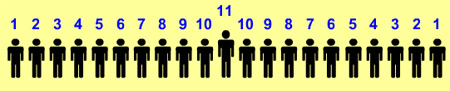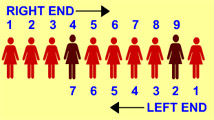# Test of Reasoning - Positions in a Group

### Score 0

1. If you are eleventh in a queue starting either end, how many are there in the queue?

Answer (c).2. In a row of 16 girls, when Hema was shifted by two places towards the left she became 7th from the left end. What was her earlier position from the right end?

Answer (b).3. In a row of children, Kavita is fourth from right and Savita is second from left. When they interchange positions Kavita is ninth from right. What will be Savita's position from left?

Answer (c).4. In a row of boys, Amrith is seventh from the left and Amar is twelfth from the right. If they interchange their positions, Amrith becomes 22nd from the left. How many boys are there in the row?

Answer (c). Amrith is 22nd from left or twelfth from right (old position of Amar). So there are 21 boys to his left and 11 boys to his right. Thus including Amrith there are 33 boys in the row.

5. In a row of girls, Clara is 9th from the left and Glory is 16th from the right. If they interchange their positions, Clara becomes 25th from the left. How many girls are there in the row?

Answer (c). Clara is 25th from left or 16th from right (old position of Glory). So there are 24 girls to her left and 15 girls to her right. Thus including Clara there are (24 + 15 + 1) = 40 girls in the row.

6. In a row of children, Ravi is fourth from right and Sham is second from left. When they interchange positions Ravi is ninth from right. What will be Sham's position from left?

Answer (c).7. Suresh is 7 ranks ahead of Ashok in the class of 39 students. If Ashok's rank is 17th from the last, what is Suresh's rank from the start?

Answer (a).8. Sudesh ranks seventh from the top and 28th from the bottom. How many students are there in the class?

Answer (a). 6 + Sudesh + 27 = 34 students in the class.

9. Ramesh ranks 13th in a class of 83 students. There are 5 students below Kishan rankwise. How many students are there between Ramesh and Kishan?

Answer (b). 12 students + Ramesh + X number of students + Kishan + 5 students = 83.
X = 83 - (12 + 5 + 2 ) = 64.

10. In a class Rajan got the 11th rank and he was 31st from the bottom of the list of boys passed. Three boys did not take the examination and one failed. What is the total strength of the class?

Answer (c). Total strength = 10 (ranked better than Rajan) + Rajan + 30 (ranked lower than Rajan) + 3 (did not take the exam) + 1 (failed) = 45

11. A is older than B but younger than C. D is younger than E but older than A. If C is younger than D, who is the oldest of all?

Answer (d). The order from the oldest to the youngest is E - D - C - A - B.

12. Among five friends A is shorter than B but taller than E, C is slightly taller than B but D is slightly shorter than B and slightly taller than A. Who is the shortest?

Answer (b). The order from the tallest to the shortest would be C - B - D - A - E.

13. There are five friends - S, K, M, A and R. S is shorter than K, but taller than R. M is the tallest. A is a little shorter than K and little taller than S. Who has two persons taller and two persons shorter than him?

Answer (d). The order from the tallest to the shortest would be M - K - A - S - R.

14. 'Somya' is shorter than 'Usha', 'Neha' is taller then 'Somya', 'Sudha' is taller than 'Usha" but shorter than 'Hema', 'Usha' is taller than 'Neha'. Who is the tallest among them?

Answer (a). The order from the tallest to the shortest would be Hema - Sudha - Usha - Neha - Somya.

15. If Sheena is taller than Meena, Reena is taller than Chitra but shorter than Beena, Meena is shorter than Chitra, Chitra is taller than Sheena, then who is the shortest?

Answer (b). The order from the tallest to the shortest would be Beena - Reena - Chitra - Sheena - Meena.

16. In a team of five players, Vani is older than Rani. Sita is younger than Vani but older than Rani. Nita is younger than Mary and Rani. Rani is older than Mary. Whose age is middle of the five in this team?

Answer (c). The order from the oldest to the youngest would be Vani - Sita - Rani - Mary - Nita.

17. Snigdha is elder than Swapna. Lavanya is elder than Swapna but younger than Snigdha. Suvarna is younger than both Hari and Swapna, Swapna is elder than Hari. Who is the youngest?

Answer (c). The order from the eldest to the youngest would be Snigdha - Lavanya - Swapna - Hari - Suvarna.

18. Lalit is elder than Prakash and Kishore. Mukesh is elder than Rakesh but not as old as Lalit. Prakash is younger than Rakesh but is not the youngest. Who is the eldest?

Answer (a). The order from the eldest to the youngest is Lalit - Mukesh - Rakesh - Prakash - Kishore.

19. Shailendra is shorter than Keshav but taller than Rakesh. Madhav is the tallest. Ashish is a little shorter than Keshav and little taller than Shailendra. If they stand in the order of increasing heights, who will be the second?

Answer (b). The order of standing in the order of increasing heights would be Rakesh - Shailendra - Ashish - Keshav - Madhav.

20. In the alphabetical order A, B, C, D, … Z, which letter is 11 th to the left of the letter which is 4 th to the right of T?

Answer (d).